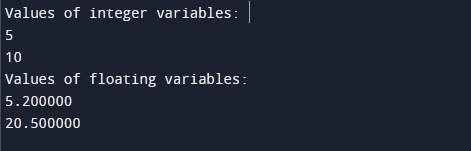# C Program for Declaring a variable and Printing Its Value

In this tutorial you will learn about the C Program for Declaring a variable and Printing Its Value and its application with practical example.

In this tutorial, we will learn to create a program where we will be declaring a variable and printing its value using C programming language.

Prerequisites

Before starting with this tutorial we assume that you are best aware of the following C programming topics:

• C Variables
• C Data Types
• C Input Output

## Program for Declaring a variable and Printing Its Value

In this program, we will declare both integer and floating type variables and print their values using printf function.

Output:-In the above program, we have first declared and initialized a set of both integer and floating type variables.

• a = integer type variable initialized with 5
• b = integer type variable initialized with 10
• c = float type variable initialized with 5.2
• d = float type variable initialized with 20.5

In the next section we have some printf statements to print values of the integer variables using integer format specifier(%d) and values of float variables using float format specifier(%f).

In this tutorial we have learn about the C Program for Declaring a variable and Printing Its Value and its application with practical example. I hope you will like this tutorial.